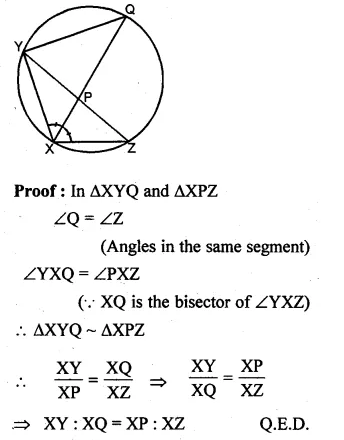## ML Aggarwal Class 10 Solutions for ICSE Maths Chapter 15 Circles Ex 15.1

These Solutions are part of ML Aggarwal Class 10 Solutions for ICSE Maths. Here we have given ML Aggarwal Class 10 Solutions for ICSE Maths Chapter 15 Circles Ex 15.1

More Exercises

Question 1.
Using the given information, find the value of x in each of the following figures :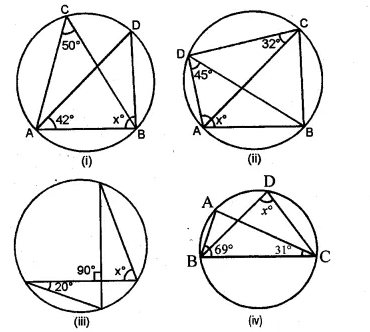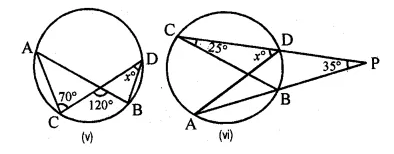Solution:
(i) ∠ADB and ∠ACB are in the same segment.
∠DAB + X + ∠ADB = 180°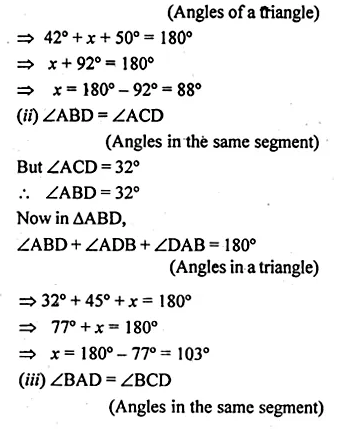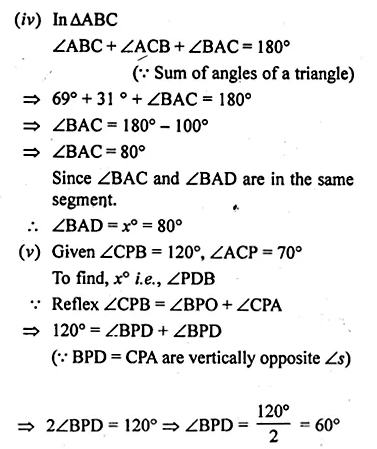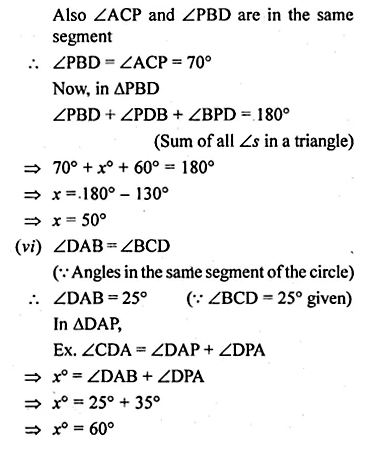Question 2.
If O is the centre of the circle, find the value of x in each of the following figures (using the given information):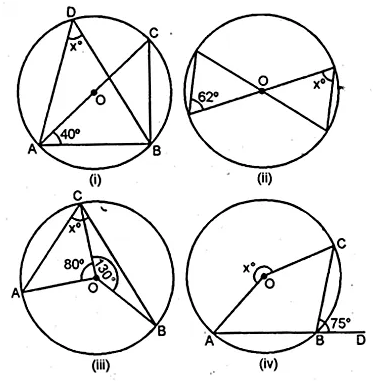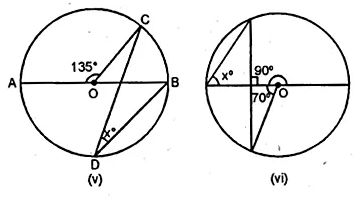Solution:
(Angles in the same segment of a circle)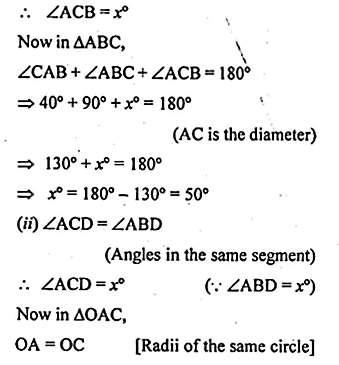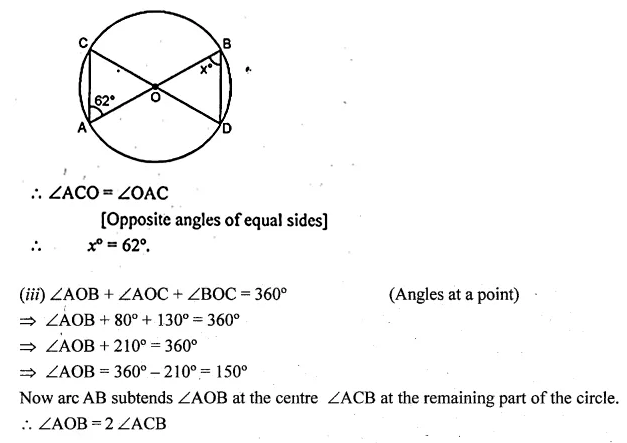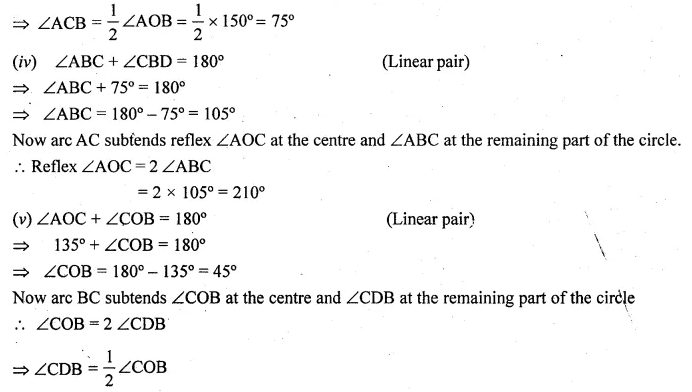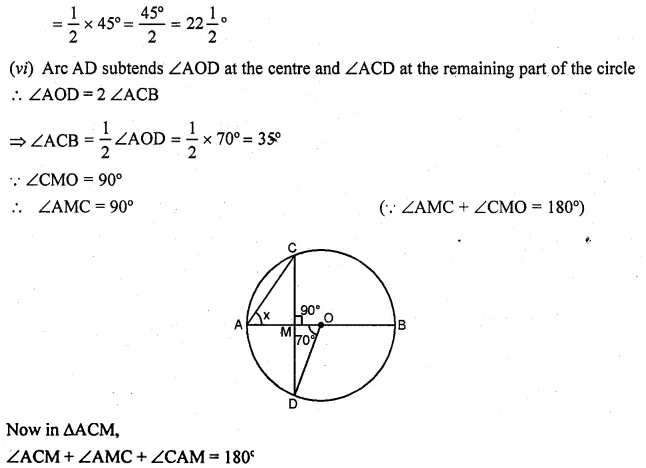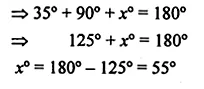Question 3.
(a) In the figure (i) given below, AD || BC. If ∠ACB = 35°. Find the measurement of ∠DBC.
(b) In the figure (ii) given below, it is given that O is the centre of the circle and ∠AOC = 130°. Find∠ABC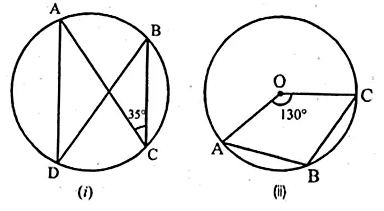Solution:
(a) Construction: Join AB
∠A = ∠C = 35° [∵ Alt angles]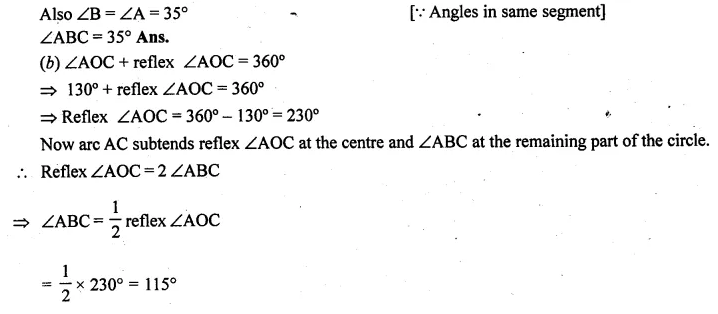Question 4.
(a) In the figure (i) given below, calculate the values of x and y.
(b) In the figure (ii) given below, O is the centre of the circle. Calculate the values of x and y.Solution:
(a) ABCD is a cyclic quadrilateral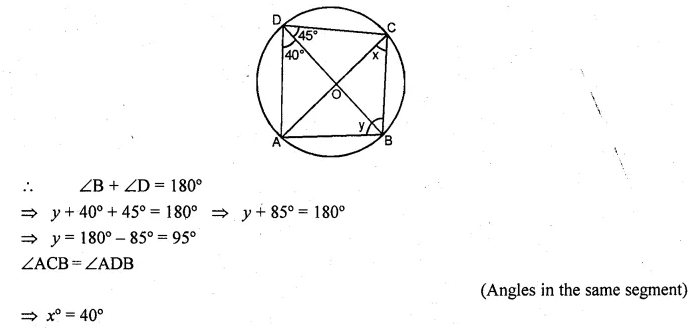Question 5.
(a) In the figure (i) given below, M, A, B, N are points on a circle having centre O. AN and MB cut at Y. If ∠NYB = 50° and ∠YNB = 20°, find ∠MAN and the reflex angle MON.
(b) In the figrue (ii) given below, O is the centre of the circle. If ∠AOB = 140° and ∠OAC = 50°, find
(i) ∠ACB
(ii) ∠OBC
(iii) ∠OAB
(iv) ∠CBA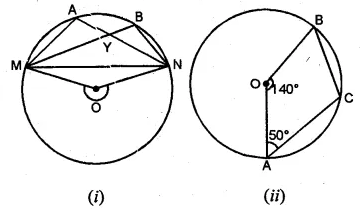Solution:
(a) ∠NYB = 50°, ∠YNB = 20°.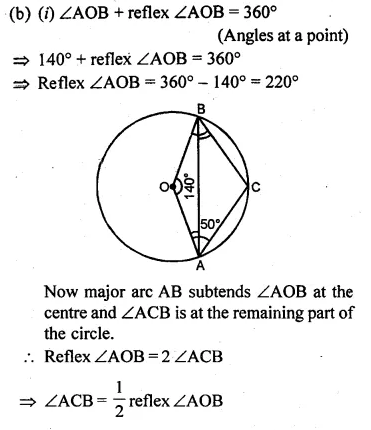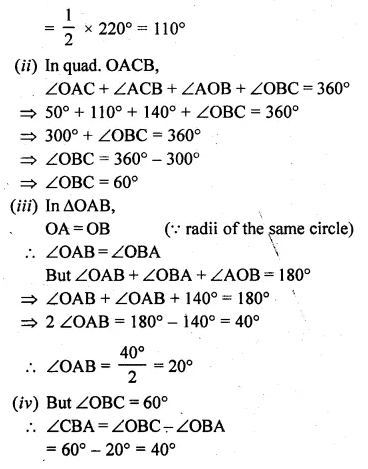Question 6.
(a) In the figure (i) given below, O is the centre of the circle and ∠PBA = 42°. Calculate the value of ∠PQB
(b) In the figure (ii) given below, AB is a diameter of the circle whose centre is O. Given that ∠ECD = ∠EDC = 32°, calculate
(i) ∠CEF
(ii) ∠COF.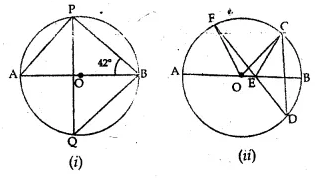Solution:
(a) In ∆APB,
∠APB = 90° (Angle in a semi-circle)
But ∠A + ∠APB + ∠ABP = 180° (Angles of a triangle)
∠A + 90° + 42°= 180°
∠A + 132° = 180°
⇒ ∠A = 180° – 132° = 48°
But ∠A = ∠PQBQuestion 7.
(a) In the figure (i) given below, AB is a diameter of the circle APBR. APQ and RBQ are straight lines, ∠A = 35°, ∠Q = 25°. Find (i) ∠PRB (ii) ∠PBR (iii) ∠BPR.
(b) In the figure (ii) given below, it is given that ∠ABC = 40° and AD is a diameter of the circle. Calculate ∠DAC.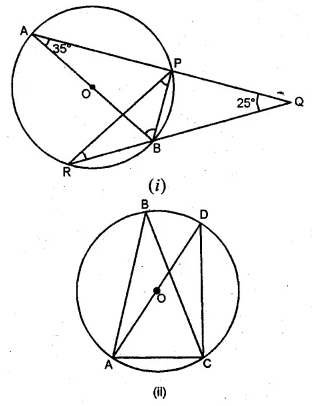Solution:
(a) (i) ∠PRB = ∠BAP
(Angles in the same segment of the circle)
∴ ∠PRB = 35° (∵ ∠BAP = 35° given)
(ii) In ∆PRQ,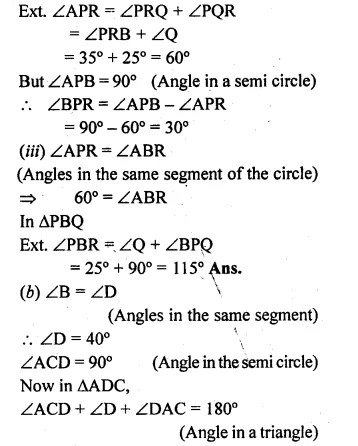Question 8.
(a) In the figure given below, P and Q are centres of two circles intersecting at B and C. ACD is a st. line. Calculate the numerical value of x.(b) In the figure given below, O is the circumcentre of triangle ABC in which AC = BC. Given that ∠ACB = 56°, calculate
(i)∠CAB
(ii)∠OACSolution:
Given that
(a) Arc AB subtends ∠APB at the centre
and ∠ACB at the remaining part of the circle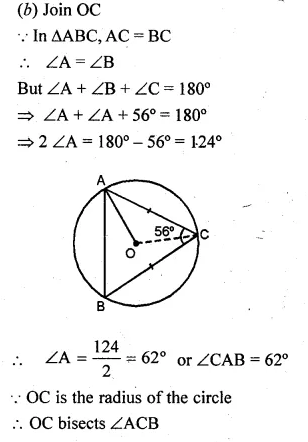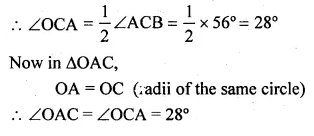Question 9.
(a) In the figure (i) given below, chord ED is parallel to the diameter AC of the circle. Given ∠CBE = 65°, calculate ∠DEC.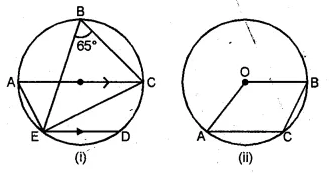(b) In the figure (ii) given below, C is a point on the minor arc AB of the circle with centre O. Given ∠ACB = p°, ∠AOB = q°, express q in terms of p. Calculate p if OACB is a parallelogram.
Solution:
(a) ∠CBE = ∠CAE
(Angle in the same segment of a circle)
⇒ ∠CAE = 65°
∠AEC = 90° (Angle in a semi circle)
Now in ∆AEC
∠AEC + ∠CAE + ∠ACE = 180° (Angle of a triangle)
⇒ 90°+ 65° +∠ACE = 180°
⇒ 155° + ∠ACE = 180°
⇒ ∠ACE = 180° – 155° – 25°
∵AC || ED (given)
∴∠ACE = ∠DEC (alternate angles)
∴∠DEC = 25°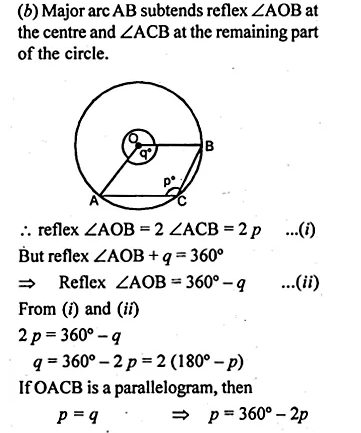Question 10.
(a) In the figure (i) given below, straight lines AB and CD pass through the centre O of a circle. If ∠OCE = 40° and ∠AOD = 75°, find the number of degrees in :
(i) ∠CDE
(ii) ∠OBE.
(b) In the figure (ii) given below, I is the incentre of ∆ABC. AI produced meets the circumcircle of ∆ABC at D. Given that ∠ABC = 55° and ∠ACB = 65°, calculate
(i) ∠BCD
(ii) ∠CBD
(iii) ∠DCI
(iv) ∠BIC.Solution:
(a) (i) ∠CED = 90° (Angle in semi-circle)
In ∆CED
∠CED + ∠CDE + ∠DCE = 180°
⇒ 90° +∠CDE + 40° = 180°
⇒ 130° + ∠CDE = 180°
⇒ ∠CDE = 180° – 130° = 50°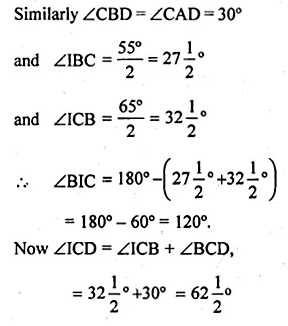NCVT MIS 2019

Question 11.
O is the circumcentre of the triangle ABC and D is mid-point of the base BC. Prove that ∠BOD = ∠A.
Solution:
In the given figure, O is the centre of circumcentre of ∆ABC.
D is mid-point of BC. BO, CO and OD are joined.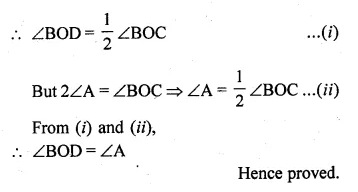Question 12.
In the given figure, AB and CD are equal chords. AD and BC intersect at E. Prove that AE = CE and BE = DE.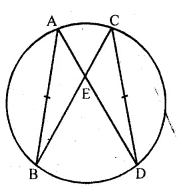Solution:
In the given figure, AB and CD are two equal chords
AD and BC intersect each other at E.
To prove : AE = CE and BE = DE
Proof:
In ∆AEB and ∆CED
AB = CD (given)
∠A = ∠C (angles in the same segment)
∠B = ∠D (angles in the same segment)
∴ ∆AEB ≅ ∆CED (ASA axiom)
∴ AE = CE and BE = DE (c.p.c.t.)

Question 13.
(a) In the figure (i) given below, AB is a diameter of a circle with centre O. AC and BD are perpendiculars on a line PQ. BD meets the circle at E. Prove that AC = ED.
(b) In the figure (ii) given below, O is the centre of a circle. Chord CD is parallel to the diameter AB. If ∠ABC = 25°, calculate ∠CED.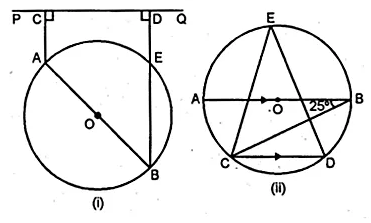Solution:
(a) Given: AB is the diameter of a circle with centre O.
AC and BD are perpendiculars on a line PQ,
such that BD meets the circle at E.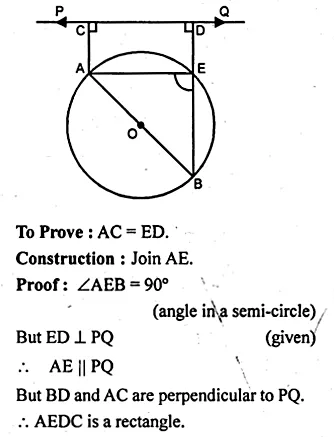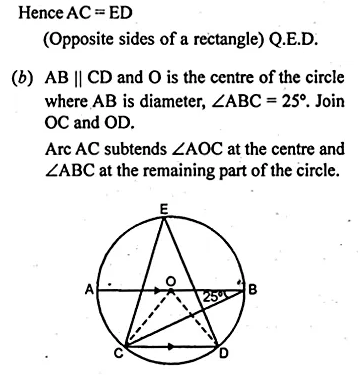Question 14.
In the adjoining figure, O is the centre of the given circle and OABC is a parallelogram. BC is produced to meet the circle at D.
Prove that ∠ABC = 2 ∠OAD.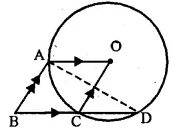Solution:
Given: In the figure,
OABC is a || gm and O is the centre of the circle.
BC is produced to meet the circle at D.
To Prove : ∠ABC = 2∠OAD.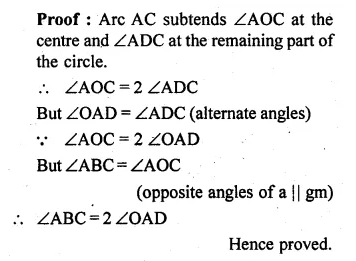Question 15.
(a) In the figure (i) given below, P is the point of intersection of the chords BC and AQ such that AB = AP. Prove that CP = CQ.(b) In the figure (i) given below, AB = AC = CD, ∠ADC = 38°. Calculate :
(i) ∠ABC (ii) ∠BEC.Solution:
(a) Given: Two chords AQ and BC intersect each other at P
inside the circle. AB and CQ are joined and AB = AP.
To Prove : CP = CQ
Construction : Join AC.
Proof: In ∆ABP and ∆CQP
∴ ∠B = ∠QQuestion 16.
(a) In the figure (i) given below, CP bisects ∠ACB. Prove that DP bisects ∠ADB.
(b) In the figure (ii) given below, BDbisects ∠ABC. Prove that $$\frac { AB }{ BD } =\frac { BE }{ BC }$$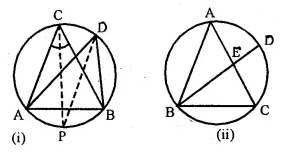Solution:
(a)Given: In the figure, CP is the bisector of
∠ACB meeting the circle at P.
PD is joined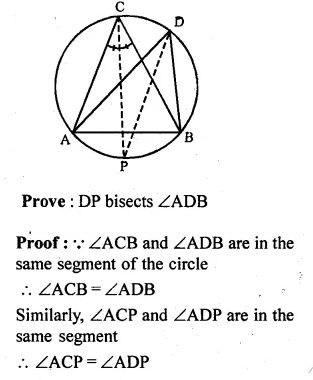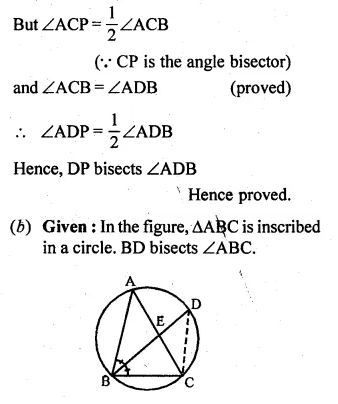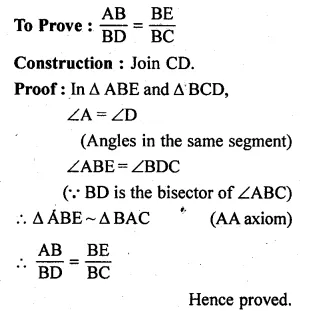Question 17.
(a) In the figure (ii) given below, chords AB and CD of a circle intersect at E.
(i) Prove that triangles ADE and CBE are similar.
(ii) Given DC =12 cm, DE = 4 cm and AE = 16 cm, calculate the length of BE.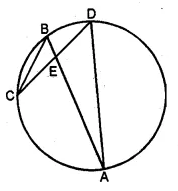(b) In the figure (ii) given below, AB and CD are two intersecting chords of a circle. Name two triangles which are similar. Hence, calculate CP given that AP = 6cm, PB = 4 cm, and CD = 14 cm (PC > PD).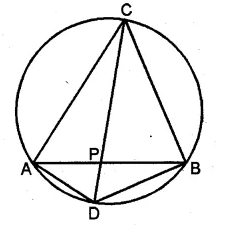Solution:
(a) Given: Two chords AB and CD intersect each other
at E inside the circle.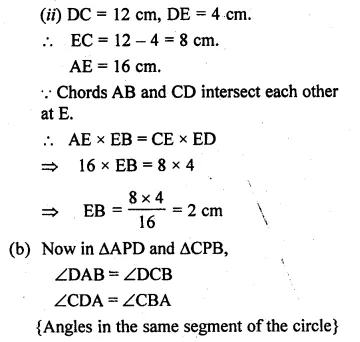Question 18.
In the adjoining figure, AE and BC intersect each other at point D. If ∠CDE = 90°, AB = 5 cm, BD = 4 cm and CD = 9 cm, find DE. (2008)Solution:
In the figure, AE and BC intersect each other at D.
AB is joined.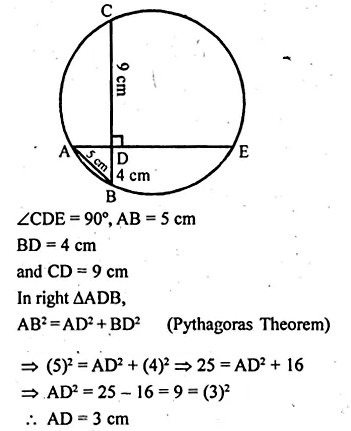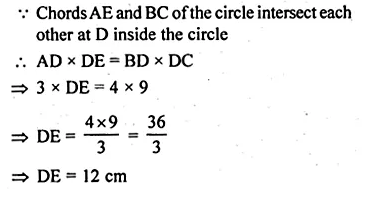Question 19.
(a) In the figure (i) given below, PR is a diameter of the circle, PQ = 7 cm, QR = 6 cm and RS = 2 cm. Calculate the perimeter of the cyclic quadrilateral PQRS.
(b) In the figure (ii) given below, the diagonals of a cyclic quadrilateral ABCD intersect in P and the area of the triangle APB is 24 cm². If AB = 8 cm and CD = 5 cm, calculate the area of ∆DPC.Solution:
(a) PR is the diameter of the circle
PQ = 7 cm, QR = 6 cm, RS = 2 cm.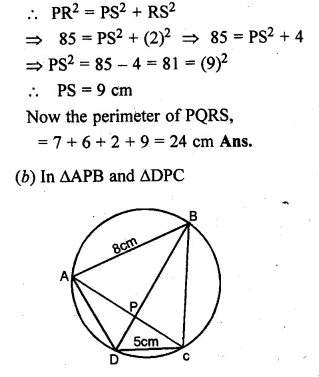Question 20.
(a) In the figure (i) given below, QPX is the bisector of ∠YXZ of the triangle XYZ. Prove that XY : XQ = XP : XZ,
(b) In the figure (ii) given below, chords BA and DC of a circle meet at P. Prove that: